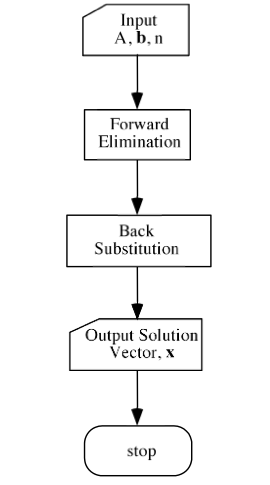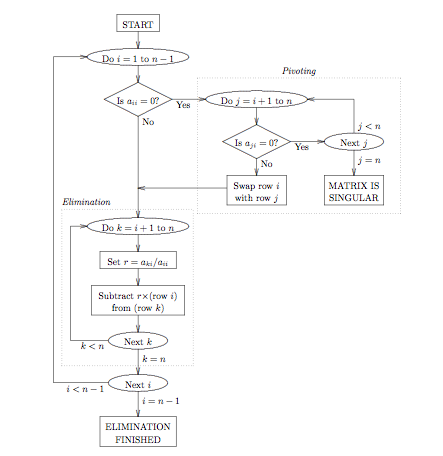# Algorithm for Gauss Elimination Method

• Start
• Declare the variables and read the order of the matrix n.
• Take the coefficients of the linear equations as:
Do for k=1 to n
Do for j=1 to n+1
End for j
End for k
• Do for k=1 to n-1
Do for i=k+1 to nDo for j=k+1 to n+1
a[i][j]=a[i][j]-a[i][k]/a[k][k]*a[k][j]
End for j
End for i
End for k
• Compute x[n]=a[n][n+1]/a[n][n]
• Do for k=n-1 to 1
sum=0
Do for j=k+1 to nsum=sum+a[k][j]*x[j]
End for j
x[k]=1/a[k][k]*(a[k][n+1]-sum)
End for k
• Display the result x[k]
• Stop

Flow Chart:[wpedon id="7041" align="center"]

## One thought on “Algorithm for Gauss Elimination Method”

1. What mean in the flowchart those intermediary OVALS? They are in fact Hexagons?